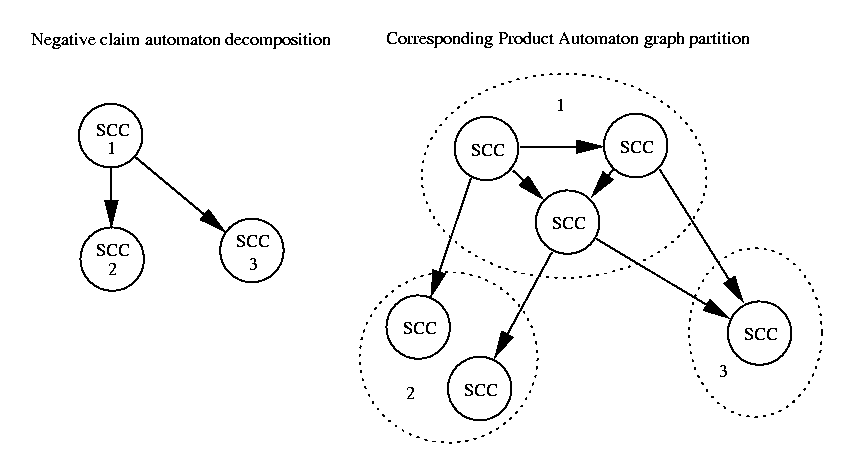# How To Distribute LTL Model-Checking Using Decomposition of Negative Claim Automaton### Jiri Barnat

barnat@fi.muni.cz

Abstract:
We propose a distributed algorithm for model-checking LTL formulas that works on a network of workstations and effectively uses the decomposition of the formula automaton to strongly connected components to achieve more efficient distribution of the verification problem. In particular, we explore the possibility of performing a distributed nested depth-first search algorithm.

LTL Model-Checking:
Model-Checking is a problem of decision whether a model of a given system satisfies a simple property expressed as a formula of some temporal logic. In the case of Linear Temporal Logic (LTL) the model-checking problem can be reduced to the problem of emptiness of Buchi automata.

To solve the problem so called Product Automaton is built. The product automaton is a result of a synchronization of the small Negative Claim Automaton with a huge System Automaton (with all states considered as accepting). The system automaton models the behavior of the given system and the Negative Claim Automaton describes the behavior which contradicts the verified property. If the language of the product automaton is not empty then the system has some illegal behavior which means that verified property is not satisfied.

Sequential Algorithmic Solution:
The standard algorithm used to solve the problem is the Nested Depth-First Search algorithm.

```PROCEDURE DFS(state)
IF (state,0) not in visited_set
THEN visited_set := visited_set + (state,0)
in_stack_set := in_stack_set + state
FOREACH s in Succ(state) DO
DFS(s)
IF Accepting(state)
THEN NDFS(state)
FI
in_stack_set := in_stack_set - state
FI
END

PROCEDURE NDFS(state)
IF (state,1) not in visited_set
THEN visited_set := visited_set + (state,1)
FOREACH s in Succ(state) DO
IF s in in_stack_set
THEN Report("Cycle")
ELSE NDFS(s)
FI
FI
END
```
Since the algorithm has to maintain all already explored states the algorithmic solution suffers from the space requirements (well known state explosion problem).

Distributed Algorithmic Solution
A possible way how to deal with the state explosion problem is the utilization of computation power of several interconnected workstations (network nodes). Unfortunately, performing the Nested DFS algorithm on several processors in parallel may result in an incorrect behavior of the algorithm. In that case the algorithm may not discover existing accepting cycle.

We can assure the correctness of the distributed Nested DFS algorithm by defining such a partition of the state space in the distributed environment that preserves locality of cycles in the Product Automaton graph and by limiting the nested part of the algorithm to those successors of a state that are local on the network node.

The key observation for an implementation is that the states of Product automaton are pairs. Each pair is made by a state of the system automaton and by a state of the Negative Claim Automaton. Hence, whenever there is a cycle C in the product automaton then there is a corresponding cycle D in the Negative Claim Automaton.

C = (s1,f1) -a1-> (s2,f2) -a2-> ... -an-> (s1,f1)

D = f1 -a1-> f_2 -a2-> ... -an-> f1

As the Negative Claim Automaton is typically small its graph can be decomposed into strongly connected components efficiently. The following picture shows the decomposition of Negative Claim Automaton and the corresponding partition of the Product Automaton graph. Each circle in the picture stands for a set of states that forms strongly connected component. Due to properties of the decomposition into strongly connected components no cycle can cross boundaries of any section in the PA graph partition.Experimental Results

 Modeled system: Elevator II Verified property formula: G(r0 -> (!p0 U (p0 U (!p0 U (p0 && o))))) && G(r1 -> (!p1 U (p1 U (!p1 U (p1 && o))))) The number of SCCs in Negative Claim Automaton decomposition: 11

 Sequential NDFS Distributed NDFS (11 nodes) Visited states 542324 maximum per node = 119773 (22%) Running time (seconds) 28.35 19.29

Conclusion
The main novelty of proposed algorithm is that we use the decomposition of the Negative Claim Automaton into maximal strongly connected components to distribute the verification problem over the network. In addition to the fact that we are able to decompose the task several instances of the verification procedure can be performed in parallel. This approach to the distribution of the algorithm can be used in the framework of multi-thread programming as well. We would like to point out that the technique is independent of the others distribution techniques, hence, it can be fruitfully combined with them.